### The 6502 overflow flag explained mathematically

The overflow flag on the 6502 processor is a source of myth and confusion. In this article, I explain signed and unsigned binary arithmetic, discuss the meaning of the overflow flag, show various formulas for computing overflow, and dispell some myths about the overflow flag.

You might be looking for my other article on overflow - The 6502 CPU's overflow flag explained at the silicon level - which is much more popular.

The 6502 is an 8-bit microprocessor that was very popular in the 1970s and 1980s, powering popular home computers such as the Apple II, Commodore PET, and Atari 400/800. The 6502 instruction set includes 8-bit addition and subtraction operations. Various status flags (carry, zero, negative, overflow) are set based on the result of the operation. Most of the flags (carry, zero, negative) are straightforward, but the meaning of the overflow (V) flag is harder to understand. If the result of a signed add or subtract won't fit into 8 bits, the overflow flag is set. (The overflag is affected in a couple other cases - the BIT operation, and the SO pin on the chip. These are discussed in detail in the excellent article The overflow flag explained, so I won't discuss them here.)

The 6502 has an 8-bit addition operation ADC (add with carry) which adds two numbers and a carry-in bit, yielding an 8-bit result and a carry out bit. The following diagram shows an example addition in binary, decimal, and hexadecimal.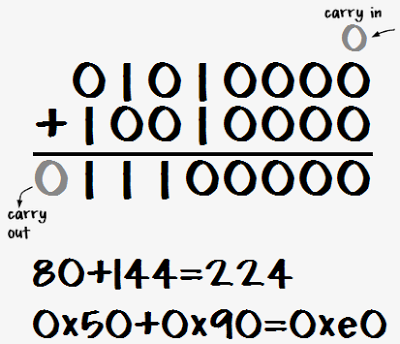The carry flag is used as the carry-in for the operation, and the resulting carry-out value is stored in the carry flag. The carry flag can be used to chain together multiple ADC operations to perform multi-byte addition.

## Ones-complement and twos-complement

The concepts of ones-complement and twos-complement are important to understand signed arithmetic. The ones complement of a number simply flips all 8 bits in the number. That is, the ones complement of N is 255-N. This is very easy to do in hardware.

The twos complement of a number is the ones complement of the number plus 1. That is, the twos complement of N is 256-N. The twos complement is very useful because adding M and the twos complement of N is the same as subtracting N from M. For example, to compute 80 - 112, simply take the twos complement of 112 (binary 10010000) and add it to 80 (binary 01010000), yielding (binary 11100000). This result is the twos complement of 32, indicating -32.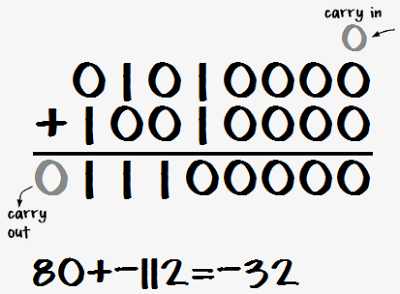Note that 80+144 and 80-112 had exactly the same bit-level operations - only the interpretation of the bits was different. This is why twos complement numbers are so useful - the same addition circuit works with them.

To see why twos complement numbers work this way, consider M + (-N) or M - N

 M - N → M - N + 256 Adding 256 doesn't change the 8-bit value. = M + (256 - N) Simple algebra. = M + twos complement of N Definition of twos complement.

Thus, adding the twos complement is the same as subtracting. (With the exception of the carry bit, which is affected by the extra 256. This will be discussed later)

## Twos-complement signed numbers

Twos complement numbers are very useful for representing signed numbers, since a number between -128 and +127 can fit into one byte: the top bit is 0 for a normal non-negative number (0 to 127), and the top bit is 1 for a twos-complement negative number (-1 to -128). (The value of the top bit is reflected in the N (negative) status flag.)

The nice thing about signed numbers is that regular binary arithmetic yields the expected results (in most cases). That is, the processor adds or subtracts the numbers as if they are unsigned binary numbers, and the right answer occurs just by interpreting them as signed.

Another example shows that the carry is ignored with signed addition. In this case, 80 and -48 are added, yielding 32. Since 80 + (256-48) = 256 + (80-48), the "extra" 256 ends up in the carry bit.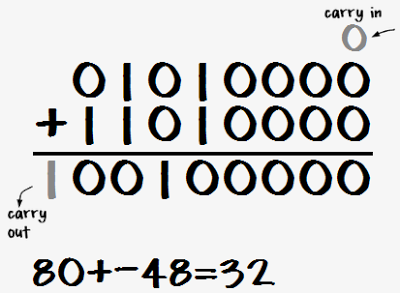Unfortunately, problems can happen. For instance, 80 + 80 = 160 with unsigned arithmetic, but with signed arithmetic the result is unexpectedly -96. The problem is that 160 will fit into a byte as an unsigned number, but it is too big to store in a byte as a signed number. Since the top bit is set, it is interpreted as a negative number. To indicate this problem, the 6502 sets the overflow flag.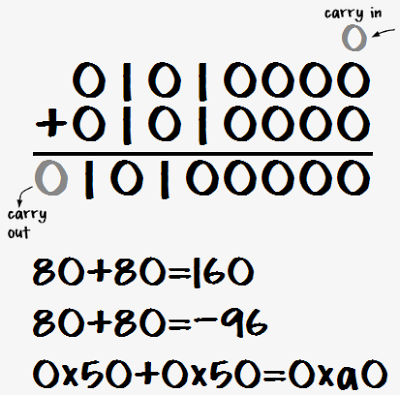## The table that explains everything about overflow

The definition of the 6502 overflow flag is that it is set if the result of a signed addition or subtraction doesn't fit into a signed byte. That is, overflow occurs if the result is > 127 or < -128. The symptom of this is adding two positive numbers and getting a negative result or adding two negative numbers and getting a positive result.

This section explores all the possible ways that overflow can occur. The following examples consider the addition of two signed numbers M and N. It is only necessary to consider the top bits of the numbers and the carry from bit 6, as shown in the diagram below, since the lower bits don't affect overflow (except by causing a carry from bit 6).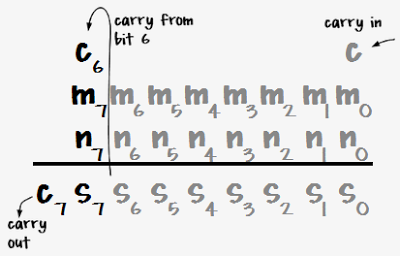There are 8 possibilities for these bits, as expressed in the table below. For each set of input bits, the table shows the carry out (C7), the top bit of the sum (S7), which is the sign bit, and the overflow bit V. This covers the 4 possibilities for sign of the arguments (positive + positive, positive + negative, negative + positive, negative + negative), with and without carry from bit 6. The table shows an example sum for each line, first expressed in hexadecimal, and then interpreted as unsigned addition and signed addition.

InputsOutputsExample
M7 N7 C6 C7 S7 VCarry / OverflowHexUnsignedSigned
000000No unsigned carry or signed overflow0x50+0x10=0x6080+16=9680+16=96
001011No unsigned carry but signed overflow0x50+0x50=0xa080+80=16080+80=-96
010010No unsigned carry or signed overflow0x50+0x90=0xe080+144=22480+-112=-32
011100Unsigned carry, but no signed overflow0x50+0xd0=0x12080+208=28880+-48=32
100010No unsigned carry or signed overflow0xd0+0x10=0xe0208+16=224-48+16=-32
101100Unsigned carry but no signed overflow0xd0+0x50=0x120208+80=288-48+80=32
110101Unsigned carry and signed overflow0xd0+0x90=0x160208+144=352-48+-112=96
111110Unsigned carry, but no signed overflow0xd0+0xd0=0x1a0208+208=416-48+-48=-96

A few interesting things can be noted from this table. Signed overflow (V=1) happens in two of the eight cases - when the result of adding two positive numbers overflows and ends up negative, and when the result of adding two negative numbers overflows and ends up positive. These rows are highlighted. Signed overflow will never happen when adding a positive number and a negative number, since the result will have a smaller magnitude. Unsigned carry (red in the unsigned column) happens in four of the eight cases, and is independent of signed overflow.

## Formulas for the overflow flag

There are several different formulas that can be used to compute the overflow bit. By checking the eight cases in the above table, these formulas can easily be verified.

A common definition of overflow is `V = C6 xor C7`. That is, overflow happens if the carry into bit 7 is different from the carry out.

A second formula simply expresses the two lines that cause overflow: if the sign bits (M7 and N7) are 0 and the carry in is 1, or the sign bits are 1 and the carry in is 0:
`V = (!M7&!N7&C6) | (M7&N7&!C6)`

The above formula can be manipulated with De Morgan's laws to yield the formula that is actually implemented in the 6502 hardware:
`V = not (((m7 nor n7) and c6) nor ((M7 nand N7) nor c6))`

Overflow can be computed simply in C++ from the inputs and the result. Overflow occurs if `(M^result)&(N^result)&0x80` is nonzero. That is, if the sign of both inputs is different from the sign of the result. (Anding with 0x80 extracts just the sign bit from the result.) Another C++ formula is `!((M^N) & 0x80) && ((M^result) & 0x80)`. This means there is overflow if the inputs do not have different signs and the input sign is different from the output sign (link).

## Subtraction on the 6502

The behavior of the overflow flag is fundamentally the same for subtraction, indicating that the result doesn't fit into the signed byte range -128 to 127. The 6502 has a SBC operation (subtract with carry) that subtracts two numbers and also subtracts the borrow bit. If the (unsigned) operation results in a borrow (is negative), then the borrow bit is set. However, there is no explicit borrow flag - instead the complement of the carry flag is used. If the carry flag is 1, then borrow is 0, and if the carry flag is 0, then borrow is 1. This behavior may seem backwards, but note that both for addition and subtraction, if the carry flag is set, the output is one more than if the carry flag is clear.

Defining the borrow bit in this way makes the hardware implementation simple. SBC simply takes the ones complement of the second value and then performs an ADC. To see how this works, consider M minus N minus borrow B.

 M - N - B SBC of M and N with borrow B → M - N - B + 256 Add 256, which doesn't change the 8-bit value. = M - N - (1-C) + 256 Replace B with the inverted carry flag. = M + (255-N) + C Simple algebra. = M + (ones complement of N) + C 255 - N is the same as flipping the bits.

The following table shows the overflow cases for subtraction. It is similar to the previous table, with the addition of the B column that indicates if a borrow resulted. Unsigned operation resulting in borrow are shown in red, as are signed operations that result in an overflow.

InputsOutputsExample
M7 N7 C6 C7 BS7 VBorrow / OverflowHexUnsignedSigned
0100100Unsigned borrow but no signed overflow0x50-0xf0=0x6080-240=9680--16=96
0110111Unsigned borrow and signed overflow0x50-0xb0=0xa080-176=16080--80=-96
0000110Unsigned borrow but no signed overflow0x50-0x70=0xe080-112=22480-112=-32
0011000No unsigned borrow or signed overflow0x50-0x30=0x12080-48=3280-48=32
1100110Unsigned borrow but no signed overflow0xd0-0xf0=0xe0208-240=224-48--16=-32
1111000No unsigned borrow or signed overflow0xd0-0xb0=0x120208-176=32-48--80=32
1001001No unsigned borrow but signed overflow0xd0-0x70=0x160208-112=96-48-112=96
1011010No unsigned borrow or signed overflow0xd0-0x30=0x1a0208-48=160-48-48=-96

Comparing the above table with the overflow table for addition shows the tables are structurally similar if you take the ones-complement of N into account. As with addition, two of the rows result in overflow. However, some things are reversed compared with addition. Overflow can only occur when subtracting a positive number from a negative number or vice versa. Subtracting positive from positive or negative from negative is guaranteed not to overflow.

The formulas for overflow during addition given earlier all work for subtraction, as long as the second argument (N) is ones-complemented. Since internall subtraction is just addition of the ones-complement, N can simply be replaced by 255-N in the formulas.

## Overflow myths

There are a lot of myths and confusion about the overflow flag. Since the flag is a bit difficult to understand, simple but wrong explanations are easy to find.

The most common myth is that just as the carry bit indicates a carry (or overflow) from bit 7, the overflow bit indicates a carry (or overflow) from bit 6 (example, example, example). As can be seen from the table above, sometimes a carry from bit 6 causes an overflow and sometimes it doesn't.

Another myth is that for multi-byte signed numbers, you use the overflow flag instead of the carry flag to carry from one byte to another (example). In fact, carry is still used to add/subtract multi-byte signed numbers, the same as with unsigned numbers.

It is sometimes claimed that the overflow bit is set if a result is too large to be represented in a byte (example, example). This omits the critical word signed - a signed result can be too large to fit in a byte, even if the unsigned result fits, and vice versa. Examples are in the table above.

Another confusing explanation is that the overflow flag is set when the sign bit is affected (example). The table shows that sometimes there is overflow when the sign bit is affected by bit 6 carry, and sometimes there is overflow when the sign bit is not affected.

## Conclusions

This is probably more than anyone really wants to know about the overflow flag. In my next article, I discuss how overflow is implemented at the silicon level.Anonymous said...

Excellent description!Anonymous said...

Excellent indeed !!! ...
Complete and shortAnonymous said...

Thank you for the very informative article. I referenced it trying to figure out a problem in my emulator. One note that might be helpful to others is that in the "Formulas for the overflow flag" section it wasn't completely clear to me that in the "(M^result) & (N^result) & 0x80" formula, the N is ones-complement of the target, not the target. It took several readings and much comprehension to give that a try.

Unknown said...

Writing a 6502 emulator. Was stuck on status flags for SBC and ADC. This was very helpful!Bob Burns said...

So I'm having some trouble. From Bruce Clarks explanation http://www.6502.org/tutorials/vflag.html

SEC ; 0 - 1 = -1, returns V = 0
LDA #\$00
SBC #\$01

I'm getting V = 1 when testing this with (n ^ m) & (n ^ o) & 0x80

but if I try 0 + -1
LDA #\$00

I get V = 0
Is Bruce Clarks explanation inaccurate? or am I missing something?

Bob

Ken Shirriff said...

Hi Bob! 0-1 should not set overflow, since -1 fits in a byte. I didn't make it super-clear in my article, but for subtraction you need to take the ones-complement of the second value before using the overflow formulas (which are designed for addition). I.e. you're converting the subtraction to addition like in your ADC example.

Hopefully this makes things more clear. If it doesn't, let me know :-)Bob Burns said...

Hi Ken. Thanks! That makes sense. Thanks for the great article.

BobPaulo said...

In the case of the SBC, shouldn't we be using the TWOS complement of N and perform an ADC, instead of ONES complement???

Bayle Shanks said...

Great article, thanks. I was wondering, in the two figures with columns "Inputs Outputs Example", the sixth rows say "...unsigned overflow..." whereas all of the other rows say "signed overflow". Is this a mistake, and if not, why is it "unsigned overflow" in that particular case?

Ken Shirriff said...

Paulo: SBC takes the ones complement and adds the carry. If the carry is 1, this is the same as the twos complement. If the carry is 0, this is the same as twos complement with borrow. The tricky part here is the carry is used as an inverted borrow flag.

Bayle: you're right; that's a typo.Anonymous said...

Nice.

And please note that the overflow bit can be set externally using the "SO" (Set Overflow) pin of the 6502 CPU and several of the variants.

Zuoliu Ding said...

This detailed explanation is very helpful to understand the overflow flag generally, including other processors.

Thanks for mentioning this simple formula nicely:
V = C6 xor C7

that would be for Intel x86 processor to implement the Overflow Flag as
OF = (carry out of the MSB) XOR (carry into the MSB)

although the 6502 CPU use another implementation here. Really enjoy reading this article, concise, understandable, and straightforward.